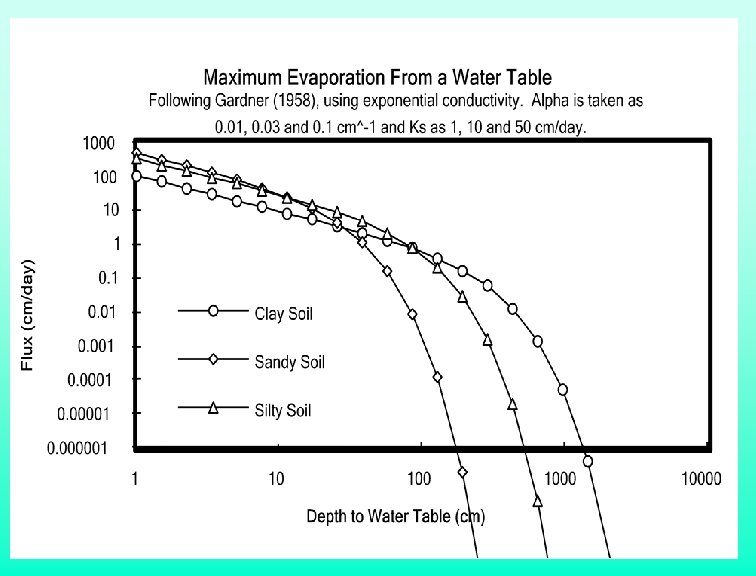# Steady Evaporation from a Water Table Following Gardner

• Slides: 17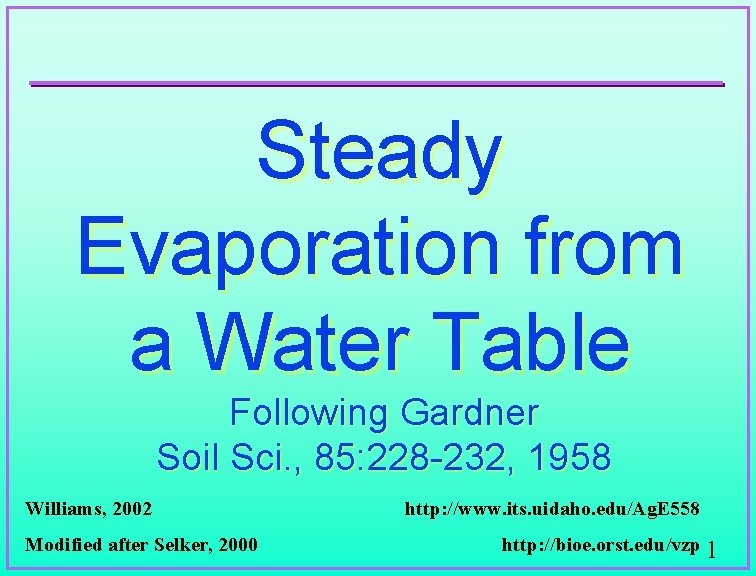Steady Evaporation from a Water Table Following Gardner Soil Sci. , 85: 228 -232, 1958 Williams, 2002 Modified after Selker, 2000 http: //www. its. uidaho. edu/Ag. E 558 http: //bioe. orst. edu/vzp 1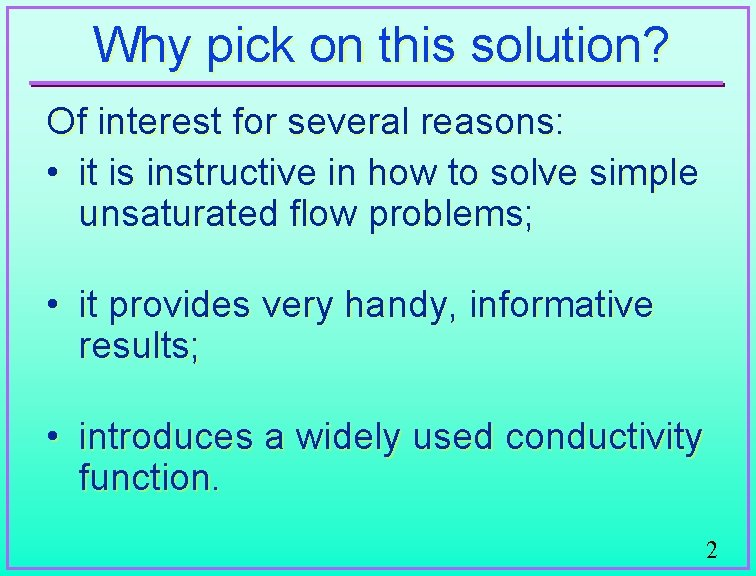Why pick on this solution? Of interest for several reasons: • it is instructive in how to solve simple unsaturated flow problems; • it provides very handy, informative results; • introduces a widely used conductivity function. 2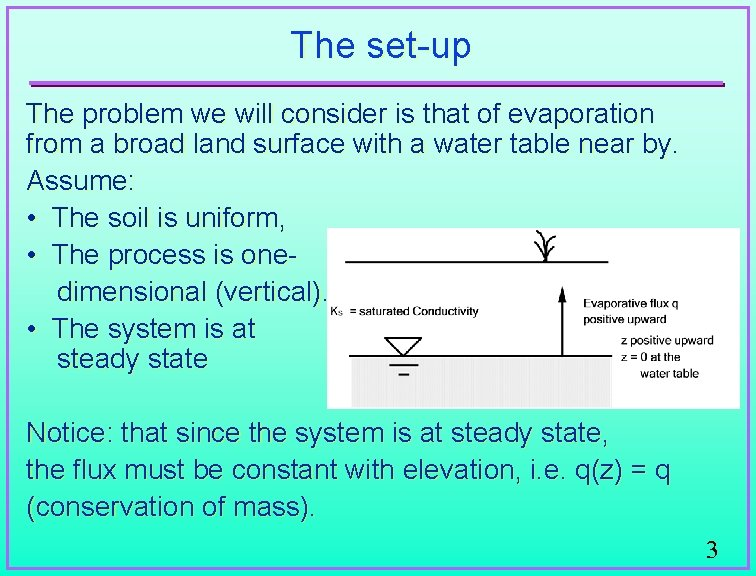The set-up The problem we will consider is that of evaporation from a broad land surface with a water table near by. Assume: • The soil is uniform, • The process is onedimensional (vertical). • The system is at steady state Notice: that since the system is at steady state, the flux must be constant with elevation, i. e. q(z) = q (conservation of mass). 3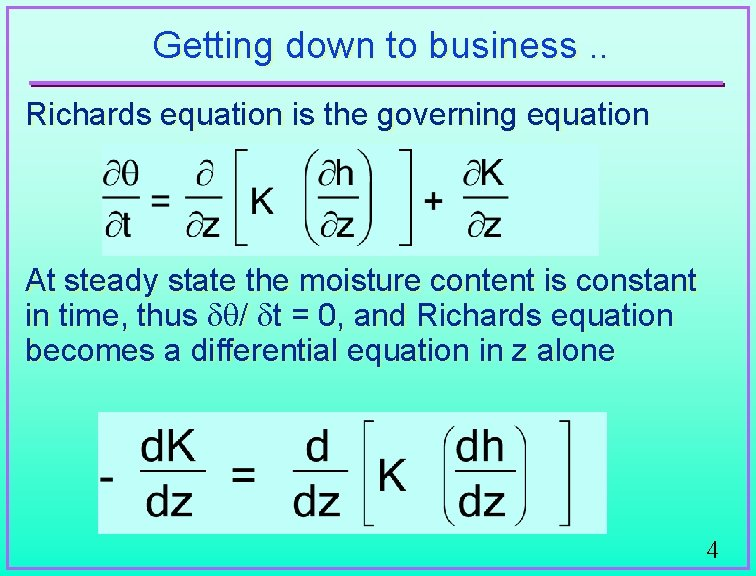Getting down to business. . Richards equation is the governing equation At steady state the moisture content is constant in time, thus d / dt = 0, and Richards equation becomes a differential equation in z alone 4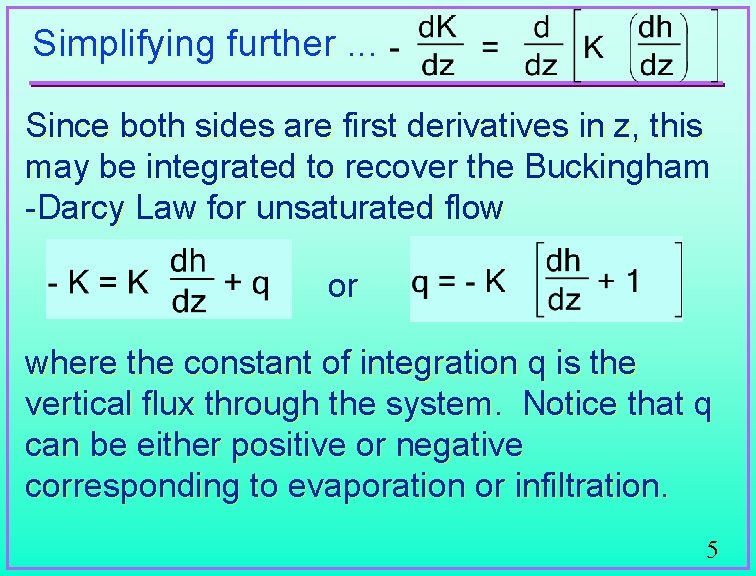Simplifying further. . . Since both sides are first derivatives in z, this may be integrated to recover the Buckingham -Darcy Law for unsaturated flow or where the constant of integration q is the vertical flux through the system. Notice that q can be either positive or negative corresponding to evaporation or infiltration. 5Solving for pressure vs. elevation We would like to solve for the pressure as a function of elevation. Solving for dz we find: which may be integrated to obtain • h' is the dummy variable of integration; • h(z), or h is the pressure at the elevation z; • lower bound of this integral is taken at the water table where h(0) = 0. 6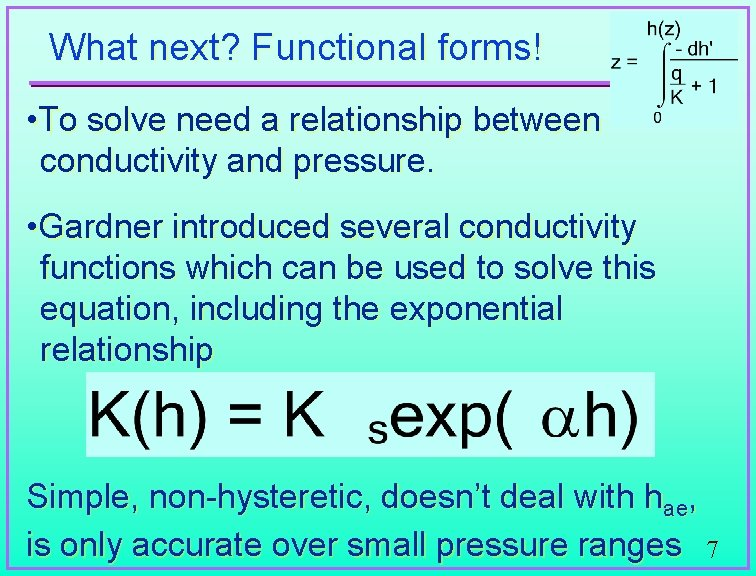What next? Functional forms! • To solve need a relationship between conductivity and pressure. • Gardner introduced several conductivity functions which can be used to solve this equation, including the exponential relationship Simple, non-hysteretic, doesn’t deal with hae, is only accurate over small pressure ranges 7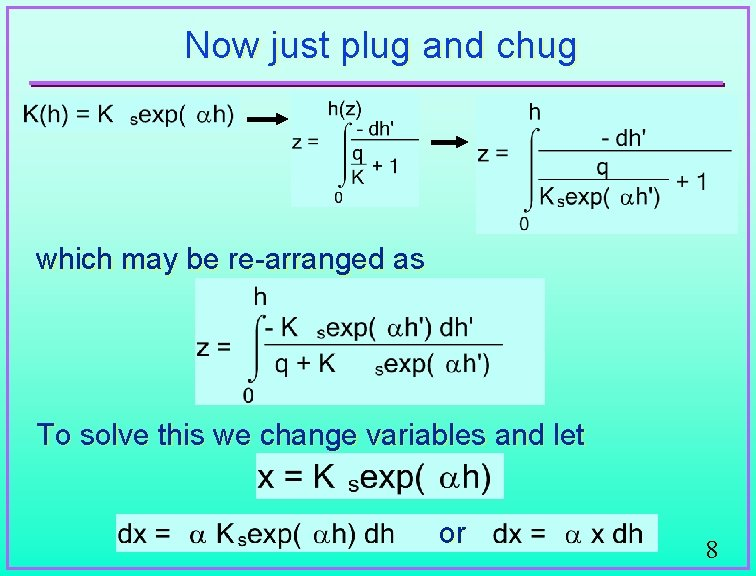Now just plug and chug which may be re-arranged as To solve this we change variables and let or 8Moving right along. . . Our integral becomes which may be integrated to obtain 9All Right! • Solution for pressure vs elevation for steady evaporation (or infiltration)from the water table for a soil with exponential conductivity. • Gardner (1958) notes that the problem may also be solved in closed form for conductivity’s of the form: K = a/(hn +b) for n = 1, 3/2, 2, 3, and 4 10Rearranging makes it more intuitive We can put this into a more easily understood form through some simple manipulations. Note that we may write: h = (1/ )Ln[exp( h)], so adding and subtracting h gives us a useful form 11We now see. . . • Contributions of pressure and flux separately. • As the flux increases, the argument of Ln[ ] gets larger, indicating that at a given elevation, the pressure potential becomes more negative (i. e. , the soil gets drier), as expected for increasing evaporative flux. • If q=0, the second term on the right hand side goes to zero, and the pressure is simply the elevation above the water table (i. e. , hydrostatic, as expected). 12Another useful form May also solve for pressure profile • Although primarily interested in upward flux, note that if the flux is -Ks that the pressure is zero everywhere, which is as we would expect for steady infiltration at Ks. 13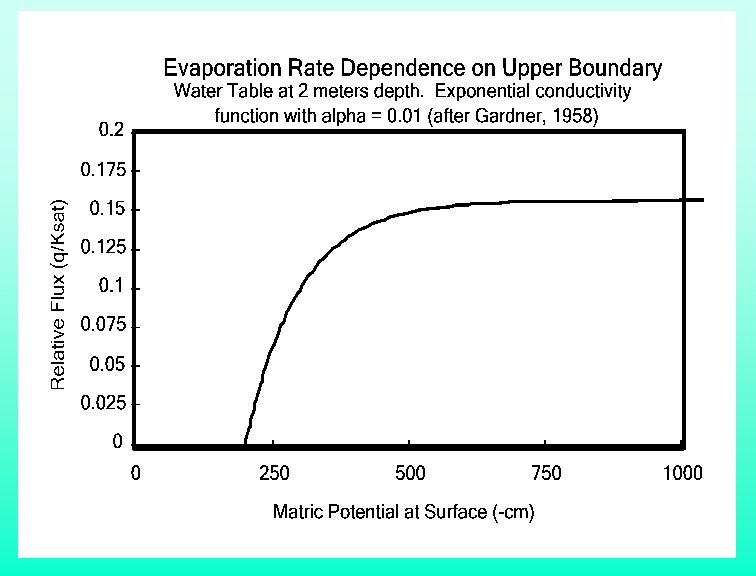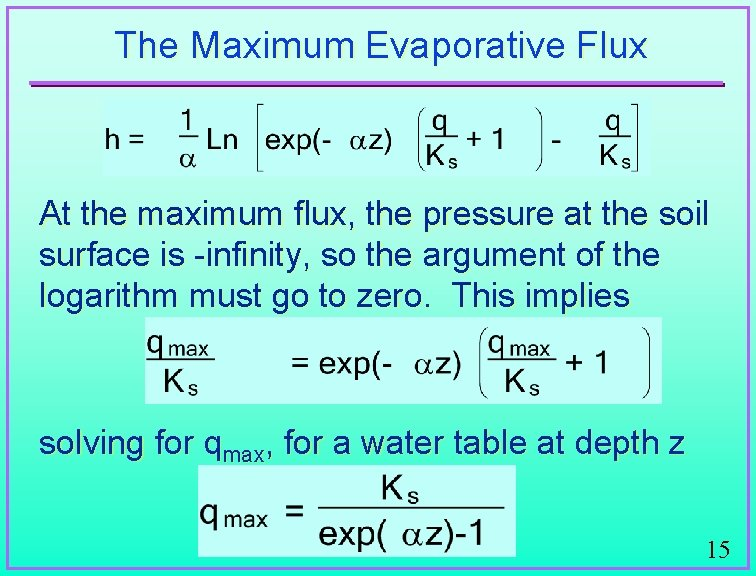The Maximum Evaporative Flux At the maximum flux, the pressure at the soil surface is -infinity, so the argument of the logarithm must go to zero. This implies solving for qmax, for a water table at depth z 15So what does this tell us? Considering successive depths of z = 1/ , 2/ , 3/ we find that qmax(z)/Ks = 0. 58, 0. 16, and 0. 05, ¨ very rapid decrease in evaporative flux as the depth to the water table increases. 16